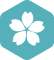### 求一公式

C/C++ > C++ 语言 [问题点数：100分，结帖人hnzlk]GitHub 绑定GitHub第三方账户获取2015年3月 C/C++大版内专家分月排行榜第二hnzlk

【线性回归】-最小二乘法一元线性回归公式推导及代码实现

1.导入依赖 import numpy as np import matplotlib.pyplot as plt 2.画出线性图 ...x = [2.8 ,2.9, 3.2 ,3.2, 3.4, 3.2, 3.3, 3.7, 3.9, 4.2] ...y = [25.3, 27.6, 29.4, 32.3, 34.1, 36.2, 35.3, 39.1, 42, 45] ...

ax2+bx+c=0ax^2+bx+c=0.(a≠0)等式两边都除以a,得, x2+bax+ca=0x^2+\frac{b}{a}x+\frac{c}{a}=0, ...方程两边都加上次项系数 ba\frac{b}{a} 的一半的平方,即方程两边都加上b24a2\frac{b^2}{4a^2},（配方）

————————————————————————————————————————————————————第篇：引入：公式的最大代数精度为2n+1，不可能达到2n+2；高斯公式的代数精度...

python利用海伦公式求三角形的面积

Gauss型公式

【Python学习笔记】6：用Gauss-Legendre公式近似积分值

Romberg(龙贝格)公式求解数值积分时的注意事项

《数值分析》第5版（李庆扬编著）的第四章课后习题第8-(2)题中，要求使用...在第步计算梯形公式时，出现了T0=pi*[f(0)+f(2pi)]。很显然，若按照sin(2pi)=sin(pi)=0计算，则Romberg(龙贝格)公式T0列前三个数

MATLAB实现一元三次方程求解/盛金公式

（海伦公式）已知三角形三条边长，面积

（海伦公式）已知三角形三条边长，面积   海伦公式：  S=（△）=√[p(p-a)(p-b)(p-c)]  其中p是三角形的周长的一半p=(a+b+c)/2. ～～～～以下转自百度百科～～～～～～～～～～～～～～～～～～～～～～～...